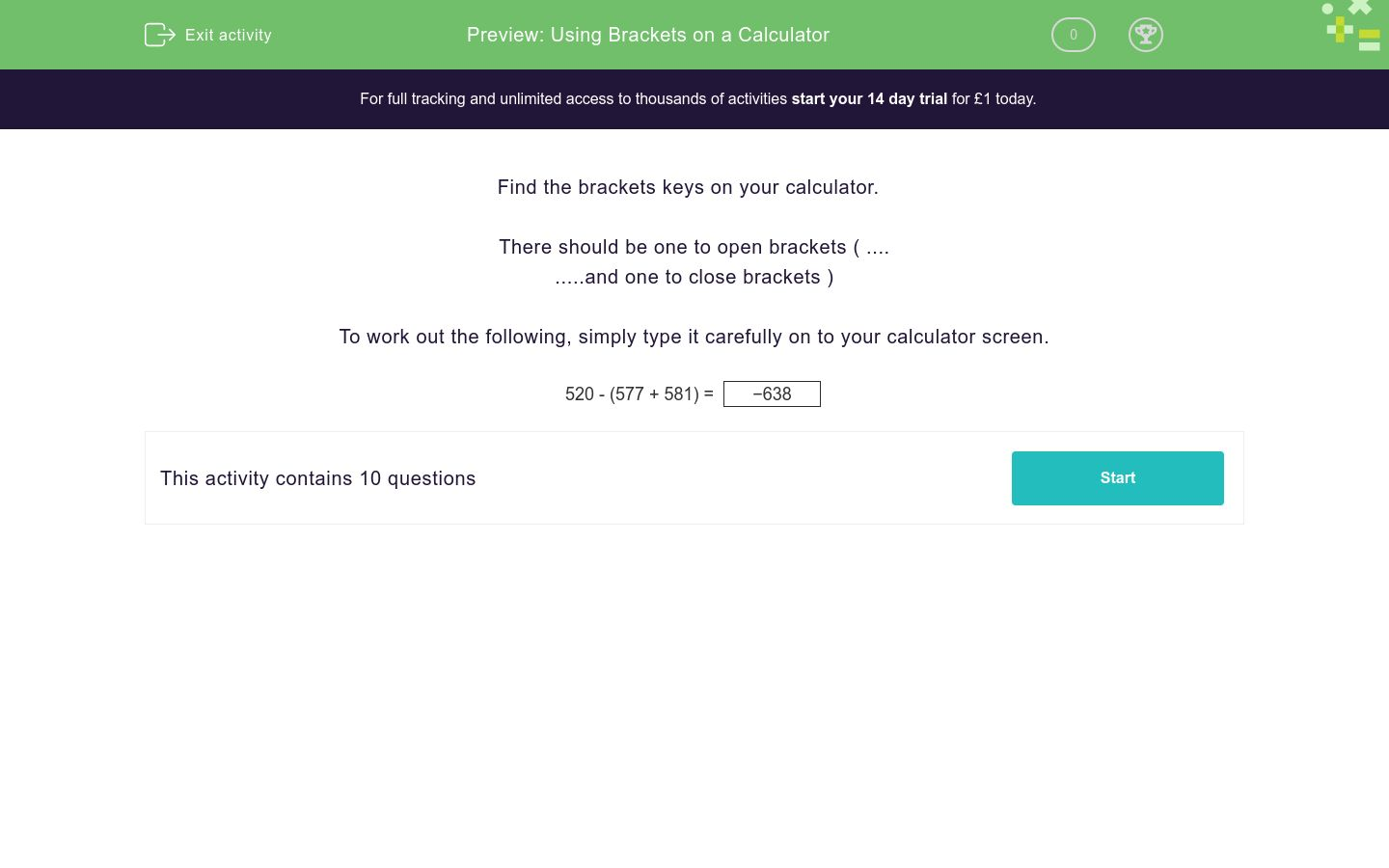# Using Brackets on a Calculator

In this worksheet, students use the brackets keys on their calculator to work out the given calculations.Key stage:  KS 3

Curriculum topic:   Number

Curriculum subtopic:   Use Calculators/Technology for Accuracy

Difficulty level:### QUESTION 1 of 10

Find the brackets keys on your calculator.

There should be one to open brackets ( ....

.....and one to close brackets )

To work out the following, simply type it carefully on to your calculator screen.

520 - (577 + 581) =
 −638

Using brackets on the calculator, work out

547 - (981 + 154) =

Using brackets on the calculator, work out

754 - (543 + 826) =

Using brackets on the calculator, work out

249 - (614 + 917) =

Using brackets on the calculator, work out

520 - (577 + 581) =

Using brackets on the calculator, work out

23 − (95.75 − 33.18) =

Using brackets on the calculator, work out

92.46 − (23.91 − 90.32) =

Using brackets on the calculator, work out

65.37 − (63.43 − 83.13) =

Using brackets on the calculator, work out

39.64 − (50.11 − 13.45) =

Using brackets on the calculator, work out

−(5822) =

Using brackets on the calculator, work out

(−5822) =
• Question 1

Using brackets on the calculator, work out

547 - (981 + 154) =
-588
• Question 2

Using brackets on the calculator, work out

754 - (543 + 826) =
-615
• Question 3

Using brackets on the calculator, work out

249 - (614 + 917) =
-1282
• Question 4

Using brackets on the calculator, work out

520 - (577 + 581) =
-638
• Question 5

Using brackets on the calculator, work out

23 − (95.75 − 33.18) =
-39.57
• Question 6

Using brackets on the calculator, work out

92.46 − (23.91 − 90.32) =
158.87
• Question 7

Using brackets on the calculator, work out

65.37 − (63.43 − 83.13) =
85.07
• Question 8

Using brackets on the calculator, work out

39.64 − (50.11 − 13.45) =
2.98
• Question 9

Using brackets on the calculator, work out

−(5822) =
-338724
• Question 10

Using brackets on the calculator, work out

(−5822) =
338724
---- OR ----

Sign up for a £1 trial so you can track and measure your child's progress on this activity.

### What is EdPlace?

We're your National Curriculum aligned online education content provider helping each child succeed in English, maths and science from year 1 to GCSE. With an EdPlace account you’ll be able to track and measure progress, helping each child achieve their best. We build confidence and attainment by personalising each child’s learning at a level that suits them.

Get started